A spring 40 mm long is stretched by the application of a force. If 10 N force required to stretch the spring through 1 mm, then work done in stretching the spring through 40 mm is

(a) 84J

(b) 68J

(c) 23J

(d) 8J

Concept Questions :-

Elastic potential energy
High Yielding Test Series + Question Bank - NEET 2020

Difficulty Level:

Two springs with spring constants ${k}_{1}$ = 1500 N/m and ${k}_{2}$ = 3000 N/m are stretched by the same force. The ratio of potential energy stored in the springs will be

1. 2:1

2. 1:2

3. 4:1

4. 1:4

Concept Questions :-

Elastic potential energy
High Yielding Test Series + Question Bank - NEET 2020

Difficulty Level:

A block of mass 2 kg moving with velocity of 10 m/s on a smooth surface hits a spring of force constant $80×{10}^{3}$ N/m as shown. The maximum compression in the spring is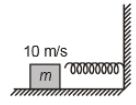1. 5 cm

2. 10 cm

3. 15 cm

4. 20 cm

Concept Questions :-

Elastic potential energy
High Yielding Test Series + Question Bank - NEET 2020

Difficulty Level:

A block of mass m = 25 kg on a smooth horizontal surface with a velocity $\stackrel{⇀}{v}$ = 3$m{s}^{-1}$ meets the spring of spring constant k = 100 N/m fixed at one end as shown in the figure. The maximum compression of the spring and velocity of the block as it returns to the original position respectively are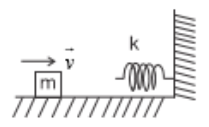1.  1.5m, -3$m{s}^{-1}$

2.  1.5m, 0$m{s}^{-1}$

3.  1.0m, 3$m{s}^{-1}$

4.  0.5m, 2$m{s}^{-1}$

Concept Questions :-

Elastic potential energy
High Yielding Test Series + Question Bank - NEET 2020

Difficulty Level:

Which of the following graph depicts spring constant k versus length l of the spring correctly

 (1)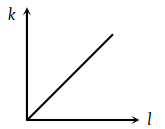(2)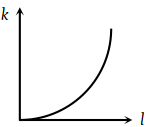(3)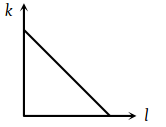(4)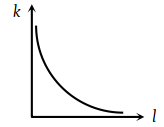Concept Questions :-

Elastic potential energy
High Yielding Test Series + Question Bank - NEET 2020

Difficulty Level:

Two springs have their force constant as k1 and ${k}_{2}\left({k}_{1}>{k}_{2}\right)$. When they are stretched by the same force

(1) No work is done in case of both the springs

(2) Equal work is done in case of both the springs

(3) More work is done in case of second spring

(4) More work is done in case of first spring

Concept Questions :-

Elastic potential energy
High Yielding Test Series + Question Bank - NEET 2020

Difficulty Level:

The potential energy of a certain spring when stretched through a distance ‘S’ is 10 joule. The amount of work (in joule) that must be done on this spring to stretch it through an additional distance ‘S’ will be:

(1) 30

(2) 40

(3) 10

(4) 20

Concept Questions :-

Elastic potential energy
High Yielding Test Series + Question Bank - NEET 2020

Difficulty Level:

A spring of force constant 800 N/m has an extension of 5cm. The work done in extending it from 5cm to 15 cm is:

(1) 16 J

(2) 8 J

(3) 32 J

(4) 24 J

Concept Questions :-

Elastic potential energy
High Yielding Test Series + Question Bank - NEET 2020

Difficulty Level:

A spring of spring constant 5 × 103 N/m is stretched initially by 5cm from the unstretched position. Then the work required to stretch it further by another 5 cm is

(1) 6.25 N-m

(2) 12.50 N-m

(3) 18.75 N-m

(4) 25.00 N-m

Concept Questions :-

Elastic potential energy
High Yielding Test Series + Question Bank - NEET 2020

Difficulty Level:

A mass of 0.5kg moving with a speed of 1.5 m/s on a horizontal smooth surface, collides with a nearly weightless spring of force constant k = 50 N/m. The maximum compression of the spring would be

(1) 0.15 m

(2) 0.12 m

(3) 1.5 m

(4) 0.5 m

Concept Questions :-

Elastic potential energy